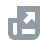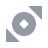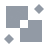Pay with
Markets
NFT
New
English
USDAccount FunctionsTutorialBinance Fan TokenBinance EarnCrypto Deposit/WithdrawalSpot & Margin TradingCrypto Derivatives
Futures Contracts
Introduction to Binance Futures
Multi-Assets Mode
USDⓈ-M Futures Contracts
COIN-M Futures Contracts
Futures Guide
Cross Collateral
Hedge Mode
Types of Orders
Binance Futures Welcome Bonus Coupon
Futures Service Agreement
Binance Futures Related Programs
Index
Portfolio Margin
Options
Leveraged TokensFinanceAPISecurityOther TopicsBinance ConvertNFTVIP

# Introduction to Binance Futures Funding Rates

2020-06-09 11:21

## .css-6hm6tl{box-sizing:border-box;margin:0;min-width:0;color:#1E2329;}1. What is Funding Rate?

Funding rates are periodic payments made to either long or short traders, calculated based on the difference between the perpetual contract prices and spot prices. When the market is bullish, the funding rate is positive and tends to rise over time. In these situations, traders who are long on a perpetual contract will pay a funding fee to traders on the opposing side. Conversely, the funding rate will be negative when the market is bearish, where traders who are short on a perpetual contract will pay a funding fee to long traders.

## 2. Why is the Funding Rate important?

The funding rate is primarily used to force convergence of prices between the perpetual contract and the underlying asset.
Unlike traditional futures, perpetual contracts have no expiration date. Thus, traders can hold positions to perpetuity unless he gets liquidated. As a result, trading perpetual contracts are very similar to spot trading pairs.
As such, crypto exchanges created a mechanism to ensure that perpetual contract prices correspond to the index. This is known as Funding Rate.

## 3. How are Funding Rates calculated on Binance?

Funding rates are calculated using the following formula:
Funding Amount = Nominal Value of Positions × Funding Rate
(Nominal Value of Positions = Mark Price x Size of a Contract)
Please note that Binance takes no fees from funding rate transfers as funding fees are transferred directly between traders.
By default, funding payments occur every 8 hours at 00:00 UTC; 08:00 UTC and 16:00 UTC for all Binance Futures perpetual contracts. In the event of extreme market volatility, Binance reserves the right to update the funding interval of a perpetual contract that differs from the default 8-hour funding interval according to the funding interval adjustment rules mentioned in Section 8 ‘Adjustment of Funding Interval’ below. Traders are only liable for funding payments in either direction if they have open positions at the pre-specified funding times. If traders do not have a position, they are not liable for any funding. If you close your position prior to the funding time, you will not pay or receive any funding.
There is a 15-second deviation in the actual funding fee transaction time. For example, when a trader opens a position at 08:00:05 UTC, the funding fee could still apply to the trader (either paying or receiving the funding fee).
You can view the Funding Rates and the countdown timer to the next funding on the Binance Futures interface above the candlestick chart:
Please note that the funding rate here represents an estimation of the last 8 hours of the premium index. For example, at 09:00 UTC, the funding rate calculation uses the premium index dataset from 01:00 to 09:00 (rather than from 08:00 to 09:00).

## 4. What determines the Funding Rate?

There are two components to the Funding Rate: the Interest Rate and the Premium. The Premium is the reason why the price of the perpetual contract will converge with the price of the underlying asset.
Binance uses a flat interest rate, with the assumption that holding cash equivalent returns a higher interest than BTC equivalent. The difference is stipulated to be 0.03% per day by default (0.01% per funding interval since funding occurs every 8 hours) and may change depending on market conditions, such as the Federal Funds Rate.
There may be a significant difference in price between the perpetual contract and the Mark Price. On such occasions, a Premium Index will be used to enforce price convergence between the two markets. The Premium Index history can be viewed here. It is calculated separately for every contract:
Premium Index (P) = [Max(0, Impact Bid Price - Price Index ) - Max(0, Price Index - Impact Ask Price)] / Price Index
Impact Bid Price = The average fill price to execute the Impact Margin Notional on the Bid Price
Impact Ask Price = The average fill price to execute the Impact Margin Notional on the Ask Price
• Price Index is a basket of prices from the major spot market exchanges, weighted by their relative volume.
• The Impact Margin Notional (IMN) for USDT-Margined Contracts is the notional available to trade with 200 USDT worth of margin (price quote in USDT); for Coin-Margined Contracts, it is the notional available to trade with 200 USD worth of margin (price quote in USD). IMN is used to locate the average Impact Bid or Ask price in the order book.
Impact Margin Notional (IMN) = 200 USDT / Initial margin rate at maximum leverage level
For example, the maximum leverage of BNBBUSD perpetual contract is 20x, and its corresponding Initial Margin Rate is 5%, then the Impact Margin Notional (IMN) is 4,000 BUSD (200 / 5%), and the system will take an IMN of 8,000 BUSD every minute in the order book to measure the average Impact Bid/Ask price.
For more information about Leverage and Margin of USDT Futures Contracts, please visit Leverage and Margin of USDⓈ-M Futures.

## 5. How to access real-time and historical Funding Rates?

You can view the real-time and historical Funding Rates by clicking [Information] - [Funding Rate History]. Alternatively, you can click here directly.

## 6. How to receive Funding Fee notifications?

1. Log in to your Binance account. Click [Derivatives] - [USDⓈ-M Futures].
2. Click on the button next to [Grid Trading] to go to [Preference].
3. Go to the [Notification] tab to toggle on the [Funding Fee Trigger] button. You can also customize the Funding Rate charges percentage between 0.0001%~0.75%. Currently, it is defaulted to 0.25%, meaning that you will be notified when the expected Funding Rate charges reach 0.25%. Click [Confirm] to save your preference.
Important note: You will be notified via email / SMS / in-app notification. This function serves as a risk warning and Binance cannot guarantee timely delivery. You agree that during your use of the Service, under certain circumstances (including but not limited to personal network congestion and poor network environment), you may not be able to receive or receive delayed reminders. Binance reserves the right and has no obligation to deliver notifications.

## 7. How to calculate the Funding Rate?

Step 1. Find the Impact Bid/Ask Price Series in a given funding period
Assume the following Bid-side order book:
If multiplier *∑px*qx > IMN in Level x and multiplier * ∑px-1*qx-1 < IMN in Level x-1, then we can find the Impact Bid Price from the Level x order book:
Impact bid price =IMN / [(IMN-multiplier *∑px-1*qx-1)/px+multiplier * ∑qx-1]
*IMN: Impact Margin Notional
To get the Impact Bid/Ask Price Series, the system performs the above methodology over the order book snapshots in this funding period:
The Ask order book is summarised as below:
*BTCUSDT perpetual contract default Impact Margin Notional
From the table above we get the following figures:
• Price at Level x-1 is 11410.50
• Accumulated quote notional quantity at Level x is 14456.38
• Accumulated base quantity at Level x-1 is：0.499 + 0.008 + 0.616 +0.079 + 0.065 = 1.267
Substituting into the formula:
Impact Ask Price = IMN / [(IMN-multiplier *∑px-1*qx-1)/px+multiplier * ∑qx-1]
= 25,000 / [(25,000 - 14456.38) / 11410.54 + 1.267]
= 25,000 / (10543.62 / 11410.54 + 1.267）
= 11,410.186 USDT
Analysis:
• The corresponding quantity when it reaches NIM at Level x: (IMN-multiplier *∑px-1*qx-1) / px = (25,000 - 14456.38 ) / 11410.54 = 0.924
• Accumulated base quantity when it reaches NIM: 0.924 + 1.267 = 2.191
• Impact Ask Price = 25,000 / (10543.62 / 11410.54 + 1.267) = 11,410.186
Step 2. Find the Premium Index Series in the observed funding period
Binance calculates the Premium Index every five seconds (12 premium index data points in a minute) and the Funding Rate is calculated by taking the time-weighted average across all 5,760 premium index data points.
Click to view the Premium Index History.
Premium Index(P) = [ Max(0, Impact Bid Price - Price Index ) - Max(0, Price Index - Impact Ask Price)] / Price Index
(Max(0,bpn-ipn)-Max(0,ipn-apn))/ipn
Step 3. Time-to-funding weighted Average of Premium Index of the observed funding period
Use the Premium Index Series in this funding period (from step 2), we substitute it to the Average Premium Index formula:
*Premium_Index_1: the first premium index data point
n = (60/5)*60*8=5760
Step 4. Calculate the Funding Rate
The Funding Rate is then calculated with this 8-Hour Interest Rate Component and the 8-Hour Premium Component. A +/- 0.05% damper is also added. For example, the Funding Rates calculated from 00:00 - 08:00 are exchanged at 08:00.
Click to view the Funding Rate History
The Funding Rate formula:
Funding Rate (F) = Average Premium Index (P) + clamp (interest rate - Premium Index (P), 0.05%, -0.05%)
*Premium Index (P) here refers to the current average
Note:
The function clamp (x, min, max) means that if (x < min), then x = min; if (x > max), then x = max; if max ≥ x ≥ min, then return x.
In other words, as long as the Premium Index is between -0.04% to 0.06%, the Funding Rate will equal 0.01% (the Interest Rate).
If (Interest Rate (I) - Premium Index (P)) is within +/-0.05% then F = P + (I - P) = I. In other words, the Funding Rate will be equal to the Interest Rate.
Example 1:
Time stamp: 2020-08-27 20:00:00 UTC
Price Index: 11,312.66USDT
Impact Bid Price: 11,316.83 USDT
Impact Ask Price: 11,317.66 USDT
Premium Index(P) = Max(0, Impact Bid Price − Price Index ) − Max(0, Price Index − Impact Ask Price) / Price Index
=Max(0, 11,316.83 - 11,312.66) - Max(0,11,312.66 - 11317.66) / 11,312.66
= (4.17 - 0) / 11,312.66
= 0.0369%
*Please note that this example is within the funding period UTC 16:00 - 24:00, the actual Premium Index at UTC 20:00 needs to be taken from time-weighted average across all indices to UTC 16:00 - 20:00 funding period.
Example 2:
Time stamp: 2020-08-28 08:00:00 UTC
Mark Price: 11,329.52
This is the end of the funding period UTC 00:00 - 08:00, 8 hours = 480 minutes, so 8-hours weighted average Premium Index(P) = 0.0429%
Premium_Index_1: the first premium index data point
Funding Rate (F) = Premium Index (P) + clamp(interest rate - Premium Index (P), 0.05%, -0.05%)
= 0.0429% + Clamp(0.01% - 0.0429%，0.05%， -0.05%)
= 0.0429% + (-0.0329%)
= 0.0100%
Step 5. Capped Funding Rate
Floor = -0.75 * Maintenance Margin Ratio
Cap = 0.75 * Maintenance Margin Ratio
Capped Funding Rate = clamp(Funding Rate, Floor, Cap)
*Funding Rate (from Step 4)
The funding rate of each contract is calculated based on its corresponding "Initial Margin Ratio" and "Maintenance Margin Ratio" at the maximum leverage level. For Initial Margin Ratio and Maintenance Margin Ratio, please refer to Leverage and Margin of USDT Futures Contracts for more detail.
For example: To calculate Floor and Cap of ADAUSDT perpetual contract, the corresponding "Initial Margin Ratio" and "Maintenance Margin Ratio" in the table at the maximum leverage level 75x is 1.3% and 0.65% respectively.

## 8. Adjustment of Funding Interval

In the event of extreme market volatility, Binance reserves the right to update the funding interval of a perpetual contract that differs from the default 8-hour funding interval. Users can visit the Real-Time Funding Rate and Funding Rate History pages to find out the latest funding interval.
Please note funding fees (if any) will be deducted from the available balance in your Futures Wallet. If you have an insufficient available balance in your Futures Wallet, the funding fees (if any) will be deducted from your position margin, which may affect your liquidation price. Please manage your positions accordingly to avoid any liquidation risks.